Want to share your content on R-bloggers? click here if you have a blog, or here if you don't.

## Background

In a previous post I was toying with better ways to integrate monoclonal proteins by deconvolution of monoclonal peaks. Turns out this is a hard problem. In any case, Deep Learning Neural Networks have become so mature that I wanted to see how easy it is to identify the presence of monoclonal proteins in the gamma region from the densitometric scan. This is not a new idea as it was first suggested in 1992 in J. Clin Pathol by Kratzer et al. I have to say, that was a very cool idea but as far as I can tell, it has not gotten much traction. This paper is mostly cited by review articles but there are a handful of further attempts. The challenges of developing a commercial product are probably regulatory in nature. However, I am hopeful that it would not be too hard to make a decision support tool.

When Kratzer et al. tackled this problem he was working on an Escom “IBM Compatible” computer with a 286 processor and 1 MB of RAM. Escom is a defunct German computer company that purchased Commodore after it began to flounder. This is why it would have been important for them to engineer their features carefully. I expect that Tensorflow may not need much feature engineering at all but I want to try what they have suggested as the features for the gamma region.

They took the scan of the gamma region, baselined it and then made it periodic and applied fast fourier transform (FFT) to represent the scan in frequency space. This is a very good idea.

## Some Basic Stuff and Sanity Checking

Let’s make a function that is a sin function for $$\ell \le x \le \ell$$ and is otherwise 0.

f1 <- function(t, l) {
if (t < -l | t > l) {
res <- 0
} else {
res <- sin(2*pi * t)
}
return(res)
}
f1v <- Vectorize(f1)

And now let’s examine this function for $$-10 < t < 10$$ and $$\ell = 1$$The FFT of this function can be determined with the R fft() function. The differential $$\Delta f$$ is calculated with the formula

$\Delta f = \frac{1}{N \Delta t}$Zooming in a bit we have:

df %>%
ggplot(aes(x = f, y = absz)) +
geom_line() +
xlim(c(0,10))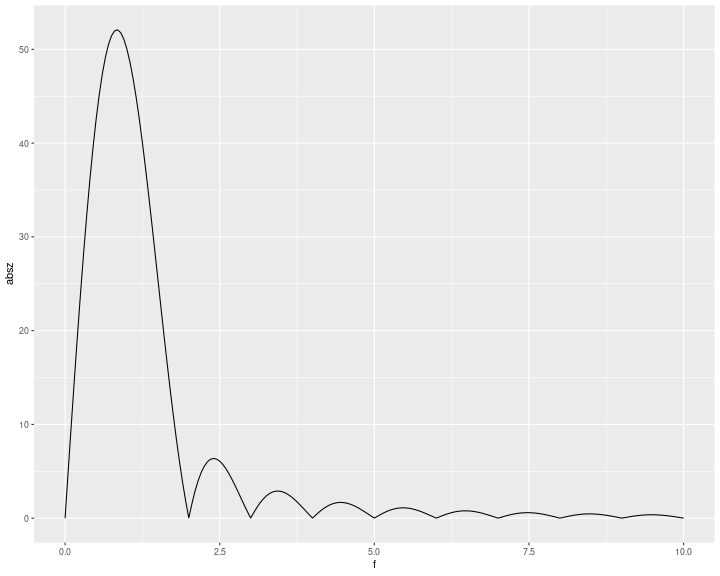This looks as it should – trying to be a delta function, namely $$\delta(x – 1)$$. As we increase the number of periods we feed the FFT the better this becomes:

df <- NULL
tmp <- data.frame(t = t, y = rep(NA,N), z = rep(NA,N), absz = rep(NA,N), f = rep(NA,N), nT = rep(NA,N))

for(i in 1:5) {
tmp <- tmp %>%
mutate(y = f1v(t, 2 * i)) %>%
mutate(z = fft(y)) %>%
mutate(absz = abs(z)) %>%
mutate(deltaf = 1 / (N * ((b - a) / N))) %>%
mutate(f = (1:N - 1) * deltaf) %>%
mutate(nT = factor(paste(2 * i, "periods"), levels = paste(2 * i, "periods")))
df <- bind_rows(df, tmp)
}

So as the number of periods increase, things improve and by 10 periods, we have a very sharp $$\delta$$ function.

df %>%
group_by(nT) %>%
ggplot(aes(x = f, y = absz)) +
geom_line() +
xlim(c(0,5)) +
facet_wrap(~nT)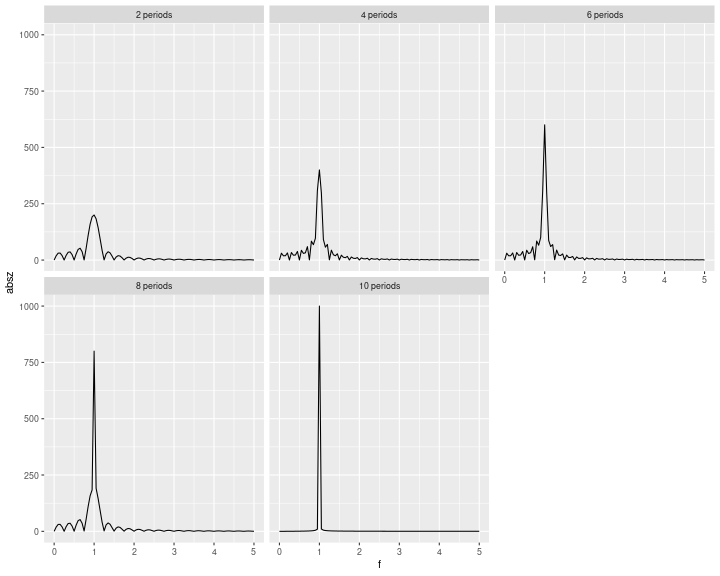## Applying FFT to a Gaussian

We can do the same thing to a Gaussian to mimic the gamma region of electrophoresis. To do this we just replicate the gamma function, inverting it in order to make the resulting function odd. Let’s just examine 5 periods for simplicity which is 10 replications of the gamma region.

library(gridExtra)
N <- 1000
a <- 0
b <- 10
t <- seq(a, b, length.out = N)

f2 <- function(x) {
remainder <- x - trunc(x)
if (ceiling(x) %% 2 == 1) {
res <- +exp(-25 * (remainder - 0.5) ^ 2)
} else {
res <- -exp(-25 * (remainder - 0.5) ^ 2)
}
return(res)
}

f2v <- Vectorize(f2)
df <- data.frame(t = t, y = rep(NA,N), z = rep(NA,N), absz = rep(NA,N), f = rep(NA,N))
df <- df %>%
mutate(y = f2v(t)) %>%
mutate(z = fft(y)) %>%
mutate(absz = abs(z)) %>%
mutate(deltaf = 1/(N*((b-a)/N))) %>%
mutate(f = (1:N - 1)*deltaf)

p1 <- df %>%
ggplot(aes(x = t, y = y)) +
geom_line() +
xlim(c(0,b))

p2 <- df %>%
ggplot(aes(x = f, y = absz)) +
geom_line() +
xlim(c(0,b))

grid.arrange(p1, p2, ncol=2)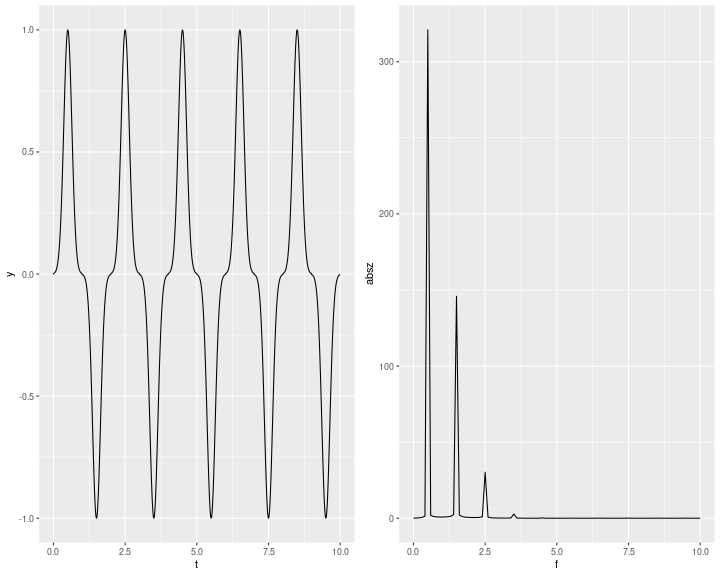So, with a pure Gaussian, we more or less have 4 peaks in the FFT. If we now mimic a monoclonal protein by adding a second Gaussian, we can see that the FFT becomes more complex because more frequencies (i.e. $$n$$) of $$e^{\frac{-2 \pi i}{N}kn}$$ are required to characterize the concatenated periodic function.

N <- 10000
a <- 0
b <- 10
t <- seq(a, b, length.out = N)

f3 <- function(x){
remainder <- x - trunc(x)
if(ceiling(x) %% 2 == 1){
res <- exp(-25*(remainder-0.5)^2) + exp(-150*(remainder-0.8)^2)
} else {
res <- -exp(-25*(remainder-0.5)^2) - exp(-150*(remainder-0.8)^2)
}
return(res)
}

f3v <- Vectorize(f3)
df <- data.frame(t = t, y = rep(NA,N), z = rep(NA,N), absz = rep(NA,N), f = rep(NA,N))
df <- df %>%
mutate(y = f3v(t)) %>%
mutate(z = fft(y)) %>%
mutate(absz = abs(z)) %>%
mutate(deltaf = 1/(N*((b-a)/N))) %>%
mutate(f = (1:N - 1)*deltaf)

p1 <- df %>%
ggplot(aes(x = t, y = y)) +
geom_line() +
xlim(c(0,b))

p2 <- df %>%
ggplot(aes(x = f, y = absz)) +
geom_line() +
xlim(c(0,b))

grid.arrange(p1, p2, ncol=2)The gamma region with a monoclonal results in an obviously more complex FFT.

## Apply to a Real Gamma Region

Here is an example densitometric scan. We can pull out the gamma region.Then we can baseline the gamma region, replicate it 10 times and apply the FFT.

library(baseline)
N <- 1000
a <- 0
b <- 10
t <- seq(a, b, length.out = N)

gamma <- filter(real_data, t > 0.6)

bl <- baseline(matrix(gamma$y, nrow = 1), method = "modpolyfit", degree = 1) gamma$y_corr <- bl@corrected[1,]
gamma$y_corr[gamma$y_corr < 0.01] <- 0
gamma$y_corr <- stats::filter(gamma$y_corr, rep(0.2,5), circular = TRUE) # force a little smoothing to get rid of cusps

#normalize the domain
gamma$t_corr <- (gamma$t - head(gamma$t,1))/(tail(gamma$t,1) - head(gamma$t,1)) gamma %>% ggplot(aes(x = t_corr, y = y_corr)) + geom_line()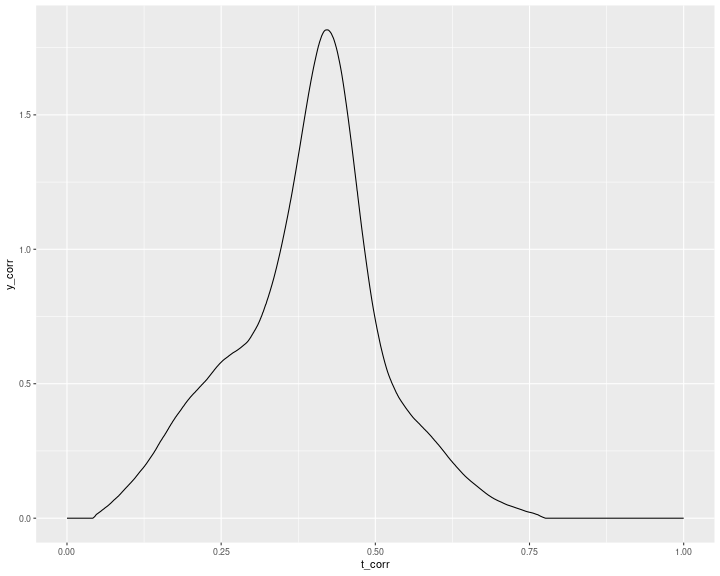# make a spline function ep_fun <- splinefun(gamma$t_corr, gamma\$y_corr)

f4 <- function(x){
remainder <- x - trunc(x)
if(ceiling(x) %% 2 == 1){
res <- ep_fun(remainder)
} else {
res <- -ep_fun(remainder)
}
return(res)
}

f4v <- Vectorize(f4)

df <- data.frame(t = t, y = rep(NA,N), z = rep(NA,N), absz = rep(NA,N), f = rep(NA,N))
df <- df %>%
mutate(y = f4v(t)) %>%
mutate(z = fft(y)) %>%
mutate(absz = abs(z)) %>%
mutate(deltaf = 1/(N*((b-a)/N))) %>%
mutate(f = (1:N - 1)*deltaf)

p1 <- df %>%
ggplot(aes(x = t, y = y)) +
geom_line() +
xlim(c(0,b))

p2 <- df %>%
ggplot(aes(x = f, y = absz)) +
geom_line() +
xlim(c(0,b))

grid.arrange(p1, p2, ncol=2)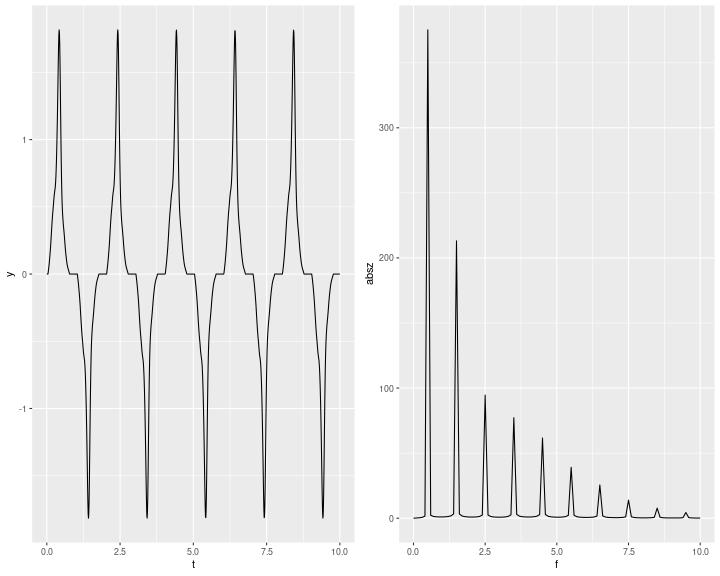And that is more or less the gist of things. The simpler the gamma region is, the fewer peaks there will be in the frequency domain. This permits recapitulation of what Kratzer et al did in their 1992 paper with modern techniques. As I say, I am not sure its necessary but it might improve model performance. Of course it would seem possible to FFT the whole scan also and reduce the dimensionality of problem to about 30 frequencies and amplitudes.

Parting Thought

As the FFT discerns the frequencies of the function, so also:

“Do not consider his appearance or his height … The Lord does not look at the things people look at. People look at the outward appearance, but the Lord looks at the heart.”

1 Sam 16:7